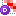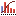AnyLogic

# Histogram 2D data

Collects data (PDF, CDF, etc.) of an array of histograms, each having a certain range of base (x) values and a range of data — y values. When an item (x,y) is added to Histogram2D Data, it first finds which individual histogram this item belongs to (this depends on the x value) and then adds y value to that histogram. The PDF and CDF are calculated for each individual histogram in the array. In addition, Histogram2D Data is capable of calculating envelopes — the areas containing a given percent of data in each simple histogram.

Histogram2D Data is particularly useful for analyzing a number of stochastic data sets, e.g. a number of realizations of a stochastic process in time obtained in different simulation runs.

The collected statistics can be visualized with the Histogram2D.

To add a histogram 2D data object

1. Drag theHistogram2D Data element from theAnalysis palette into the graphical editor.
2. Go to the Properties view.
3. Specify the expression, that will be dynamically evaluated to obtain the current Y-value of the histograms, in the Vertical axis value property.
4. If you want this histogram to store time samples as base (x axis) values, select the Use time as horizontal axis value checkbox. Otherwise, specify the expression, that will be dynamically evaluated to obtain the current base (X) value of the histograms, in the Horizontal axis value property.
5. Specify horizontal and vertical intervals for each histogram in the X-axis values range and Y-axis values range sections of properties correspondingly.
6. If you want to calculate and display envelopes — you may specify comma-separated list of lower bounds of envelopes (the areas containing a given percent of data) in the Envelopes property.
7. Finally, choose, how you want this data element to be updated.

## Properties

General

Name — The name of the histogram 2D data. The name is used to identify and access this analysis data object.

Ignore — If selected, this analysis data object is excluded from the model.

Visible — If selected, the analysis data object is visible on a presentation at runtime.

Show name — If selected, the name of this analysis data object is displayed on a presentation diagram.

Use time as horizontal axis value — If selected, the histogram 2D data element is timed, i.e. when new samples are added to the histogram 2D data, Y-value is evaluated using the specified Vertical axis value expression, while X-value takes the current model time value.
Otherwise, the histogram 2D data element is phased, i.e. both X- and Y- values of the histograms are evaluated using the specified expressions (Horizontal axis value and Vertical axis value correspondingly).

Horizontal axis value — [Enabled if Use time as horizontal axis value is not selected] Expression, that will be dynamically evaluated to obtain the current base (X) value of the histograms.

Vertical axis value — Expression, that will be dynamically evaluated to obtain the current Y-value of the histograms.

Envelopes — Specify here comma-separated list of lower bounds of envelopes — the areas containing a given percent of data.

X (Y) axis values range

Number of intervals — The number of intervals for the X (Y) axis.

Range, from ... to — Here you define the range for X (Y) axis values.

Data update

Do not update data automatically — If selected, histogram2D data are not updated automatically. In this case you should add new samples by yourself as described in Updating analysis data objects.

Update data automatically — If selected, new data samples are added automatically with the specified Recurrence time. Also, you can define here whether you want to Use model time or Use calendar dates. Depending on this choice, you can specify when updating begins with either First update time or Update date properties.

## API for working with collected data

The Histogram 2D Data element is represented in AnyLogic with instance of the class Histogram2DData This class provides a number of functions described below.

Functions
Function Description
void add(DataSet dataset) Adds the whole contents of a given dataset to the histogram data, updates PDF, CDF, and count.
void add(double xval, double yval) Adds a sample data item to the histogram data, updates PDF, CDF, and count.
int count(int xindex) Returns the number of samples added to the histogram with xindex.
double getCDF(int xindex, int yindex) Returns the CDF (cumulative distribution function) at the END of the interval yindex of the histogram with xindex.
int getNumberOfXIntervals() Returns the number of base (x) intervals, i.e. the number of individual histograms.
int getNumberOfYIntervals() Returns the number of data (y) intervals in each individual histogram.
double getPDF(int xindex, int yindex) Returns the PDF (probability distribution function) of the histogram with xindex at the interval yindex.
double getPDFOutsideHigh(int xindex) Returns the percent of samples (0..1) in the histogram xindex higher than the specified maximum.
double getPDFOutsideLow(int xindex) Returns the percent of samples (0..1) in the histogram xindex lower than the specified data (y) minimum.
double getXMax() Returns the maximum x (base) value.
double getXMin() Returns the minimum x (base) value.
double getYMax() Returns the maximum y (data) value.
double getYMin() Returns the minimum y (data) value.
void reset() Fully resets the histogram data: discards all PDF/CDF data and statistics.
void setEnvelopes(double[] env) Sets the array of envelopes to calculate.
String toString() Returns a tab-separated multi-line textual representation of the histogram data.